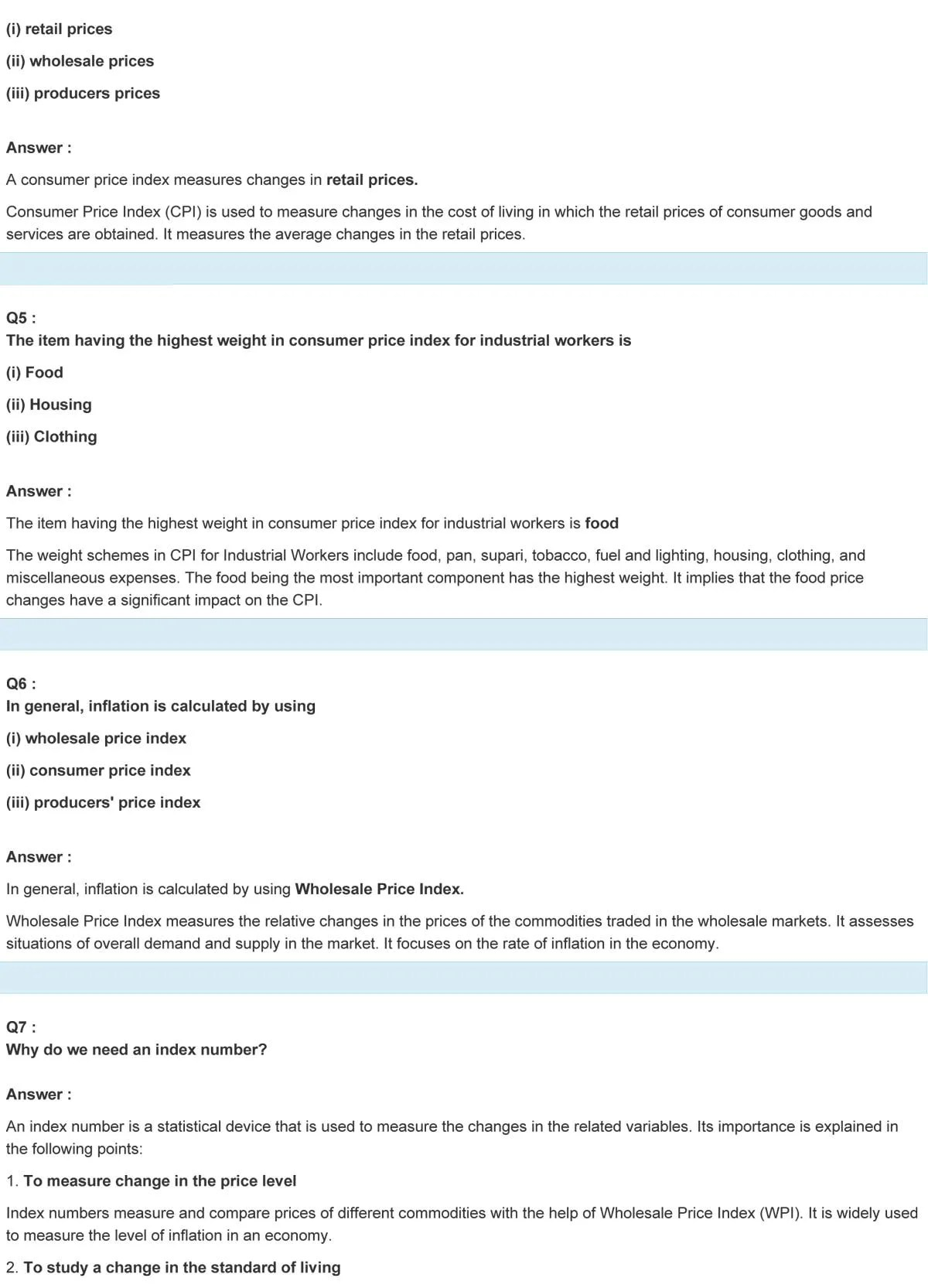# NCERT Solutions For Class 11 Chapter 8 Statistics

### NCERT Solutions Class 11 Statistics Chapter 8

NCERT Solutions are known to be a helpful book while studying for the CBSE Class 11 Statistics examinations. This study material possess an intense knowledge and the solutions collected by the subject matter experts are beneficial for the students.

NCERT Solutions Class 11 Statistics Chapter 8 Index Numbers provides us with the useful data to all the topics. These NCERT books assists the students to score well in their examinations. The students have to learn the basic fundamentals of the subject Statistics in class 11; NCERT books explains all the concepts in a comprehensive way.

## Concepts covered in this chapter –

• Introduction
• What Is An Index Number?
• Construction of an index number
• The Aggregative Method
• Method of Averaging relatives
• Consumer price index

Class 11 NCERT solutions for Statistics chapter 8 provides a wide degree of illustrative examples; which helps the students to understand and learn quickly. For more solutions and study materials Statistics, refer NCERT solutions class 11 Statistics.